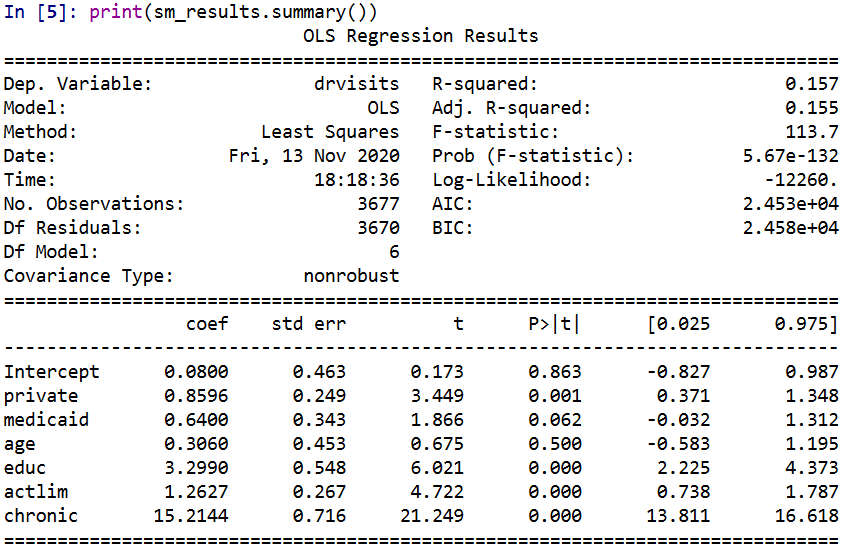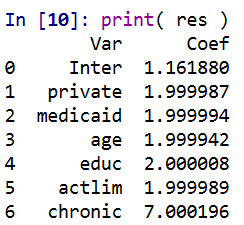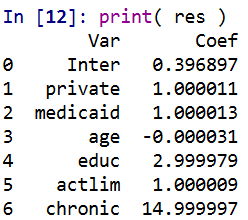# Regression with Simple Weights

I was reminded of this paper by Jung et al. on constructing simple rules via regression recently. So in the past few posts I have talked about how RTM (1,2) is aimed at making simple models. This is via variable selection and/or simplying the inputs to be binary yes/no. But in the end the final equation could be something like:

``log(Crime) = -0.56 + 0.6923*NearbyBars + 0.329*HighDensity311``

The paper linked above is about making the regression weights simple, so instead of a regression weight of 0.89728, you may just round the regression weight to 1. The Jung paper does a procedure where they use lasso regression and then round the weights. But there is a simpler approach IMO I will illustrate, just amend the lasso weights to push the coefficients to simple integers. (Also reminded by this example of using an iterative linear program to push weights to binary 0/1.)

So in lasso, you estimate your normal regression equation, but put a penalty on the weights that is typically something like `lambda*(sum(abs(reg_weights)) - 1)**2`. So if you have reg weights that add to more than 1, they are penalized by a particular amount (the lambda is a tuner to make the penalty higher/lower). And in the iterative algorithm to minimize your loss function plus this added penalty, it will converge to regression weights that meet the criteria of in total summing to around 1. Not exactly 1 but close.

You can however swap out that penalty term with whatever you want (or add to it additional penalties). I will show an example of using a penalty term to push regression coefficients towards integer values, creating simple regression weights.

# Why Simple Models?

Dan Simpson has a good blog post of the Jung paper and why simple models are sometimes preferable (and I also have a comment why simple models like this tend to work out well for CJ datasets). But here are few quick examples why you might want a simple model results.

Example 1: If you have people in the field who are tabulating data and making quick decisions, it may be they need to use pen/paper and make a quick decision. No time to input results into a computer and pop out a prediction. Imagine a nurse in the ER, or even your general practitioner. There may be quite a bit of utility in making a simple check list that says `if +4 on this scale, do a more intensive treatment`.

Example 2: You have a complicated, large database. It is easier to create a simple predictive model in SQL to serve up predictions (either because of latency or because of the complexity of the data pipeline). Instead of a complicated random forest, a linear regression with simple weights will be much easier to implement.

Example 3: Transparency. Complicated models are more difficult to understand and monitor. If you have a vested interest in presenting the model to outside parties, it may make sense to sacrifice some accuracy to make the model more interpretable. Also similar to lasso, I suspect these simple weights will reduce the variance of predictions.

The reason that these simple weights work well in practice for many social science examples you could interpret either in a good light or a bad one. For the half-empty interpretation, our models are not well identified – we can literally swap out various weights in our regression equation and get near similar predictions. So it is fools errand to try to find the regression equation that describes the underlying system. But you can flip that around as well, we don’t even need to find the perfect equation, we can identify quite a few good predictive equations. And why not pick a good equation that is easier to interpret?

# Pytorch Example

The example set of code here is very simple, so I will just put the python code entirely in this post. First I import my libraries I am using and change my directory.

``````############################################
import os
import torch
import statsmodels.api as sm
import statsmodels.formula.api as smf
import pandas as pd
import numpy as np

my_dir = r'D:\Dropbox\Dropbox\Documents\BLOG\regression_simpleweights\analysis'
os.chdir(my_dir)
############################################``````

Next I read in the data, which I have previously used as an example in prior blog posts on doctor visits for medicare patients. One thing to note here, is that I rescale the independent variables I am using to min/max. So the age variable instead of going from 65-90 like in the original data, now is scaled to be between 0/1. This is a problem intrinsic to lasso as well, in that you can change the scale of the input variables and it changes the weights. Here with the original data, the education variable has a tiny regression coefficient (0.2), but is highly stat significant. So without rescaling that variable, the model said to hell with your penalty and still converged to a solution of that regression weight is 0.2. If you divide the education variable by 5 though, the corresponding regression weight would change to around 1.

``````###########################################
#Data from Stata, https://www.stata-press.com/data/r16/gsem_mixture.dta
#see pg 501 https://www.stata.com/manuals/sem.pdf

y_dat = visit_dat[['drvisits']]
x_vars = ['private','medicaid','age','educ','actlim','chronic']
#rescaling variables to 0/1
x_dat = visit_dat[x_vars]
visit_dat[x_vars] = (x_dat - x_dat.min(axis=0)) / ( x_dat.max(axis=0)  - x_dat.min(axis=0) )
x_dat = visit_dat[x_vars] #intentional not a copy
###########################################``````

Now in the next part, I estimate the default linear regression model using statsmodels for reference. Then I stuff the results into pytorch tensors (which I will use later as default starting points for the pytorch estimates). Below is a pic of the resulting summary for the regression model (with the scaled variables, so is slightly different than my prior post).

``````###########################################
#Estimating the same model in statsmodel
#for confirmation of the result

stats_mod = smf.ols(formula='drvisits ~ private + medicaid + age + educ + actlim + chronic',
data=visit_dat)
sm_results = stats_mod.fit()
print(sm_results.summary())

#What is the mean squared error
pred = sm_results.get_prediction().summary_frame()
print( ((y_dat['drvisits'] - pred['mean'])**2).mean() )
#169513.0122252265 for sum
#46.10 for mean

#for setting default initial weights
coef_table = sm_results.params
###########################################``````Now creating the pytorch model is quite simple. For linear regression it is just one linear layer, and then setting the loss function to mean squared error. Then I create my own simple weight penalization factor in the `simp_loss` function. This takes the regression weights (not including the bias/intercept term), takes the difference between the observed weight and the rounded weight, takes the absolute value and sums those absolute values up. Then in the loop when I am fitting the model, you can see the `loss = criterion(y_pred, y_ten) + 0.4*simp_loss(model)` line. For the usual linear regression, it would just be the first criterion term. Here to add in the penalty term is super simple in pytorch, you just add it to the loss. (And you can incorporate additional penalities, the same way ala elastic-net. The Jung paper they put a penalty on the sum of coefficients as per the original lasso as well.)

Then the final part of the code after the loop is just putting the coefficients in a nicer data frame to print. And below the code snippet are the results.

``````###########################################
#Now estimating OLS model with simple coefficient
#Penalities in Pytorch

torch.manual_seed(10)

model = torch.nn.Sequential(
torch.nn.Linear(len(x_vars),1,bias=True)
)

##Initializing weights
#    model.weight = torch.nn.Parameter(coef_ten)
#    model.bias = torch.nn.Parameter(int_ten)

x_ten = torch.tensor( x_dat.to_numpy(), dtype=torch.float)
y_ten = torch.tensor( y_dat.to_numpy(), dtype=torch.float)

criterion = torch.nn.MSELoss(reduction='mean')

def simp_loss(mod):
dif = mod.weight - torch.round(mod.weight)
return dif.abs().sum()

for t in range(100000):
#Forward pass
y_pred = model(x_ten)
#Loss
loss = criterion(y_pred, y_ten) + 0.4*simp_loss(model)
if t % 1000 == 99:
print(f'iter: {t}, loss: {loss.item()}')
loss.backward()
optimizer.step()

#Making a nice dataframe of coefficients

coef_vars = ['Inter'] + x_vars
vals = list(model.bias.detach().numpy()) + list(model.weight.detach().numpy()[0,:])
res = pd.DataFrame(zip(coef_vars, vals), columns=['Var','Coef'])
print( res )
###########################################``````Here I did not round the coefficients, so you can see that they are not exactly integer values, but are very close. So this will result in a lower loss than taking the usual linear regression coefficients and rounding them like in the noted Jung paper. It is a more direct approach. Also note that the intercept is not close to an integer value. I did not include the intercept in my penalty term. You could if you wanted to, but for most examples I don’t think it makes much sense to do that.

But one of the things that I have noticed playing around with pytorch more is that it is very difficult to get random initialized weights to converge to the same solution. That identification problem I mentioned earlier. One way is instead of using random initialized weights, is to initialize them to some reasonable values. If you uncomment the lines `with torch.no_grad():` in the above code and initialize the weights to start from the unregularized OLS solution, it converges much faster, has a slightly smaller mean square error term, and results in these effects:So you can see in that solution it is exactly the same as rounding the initial OLS solution (ignoring the intercept again). But that may not always be the case. For example if actlim (activity limitations) and educ (education) had a very high correlation, it may be rounding both down is too big a hit to the fit of the equation, so one may go down and one go up. (You need to estimate the equation to know if things like that will occur.)

And that is all folks! While if I were sharing this more broadly, I would likely make a statsmodel like interface (and it appears they use cvxopt under the hood) instead of pytorch, it is very simple to amend pytorch to return simple weights, just add in the penalty to the loss function. Works the same way for lasso/ridge as it does for the simple weights example I give here.

Next up I want to try to figure out autograd in pytorch good enough to give standard errors for these various regression models I am estimating. While I don’t think hypothesis testing makes sense for these various models I am sharing, seeing a standard error that is very high may have prognostic value. In this case, if you had a very high standard error relative to the simple coefficient, it might suggest you should rescale the variable a different way or drop it entirely.

Also for this example, to be simple in the field it would not only need simple coefficients, but simple inputs as well. Wondering if there is a way to add in threshold layers in deep learning to automatically figure out the best way to make the inputs binary (e.g. above 70, educ below 10, etc.) instead of doing min/max scaling of the inputs.